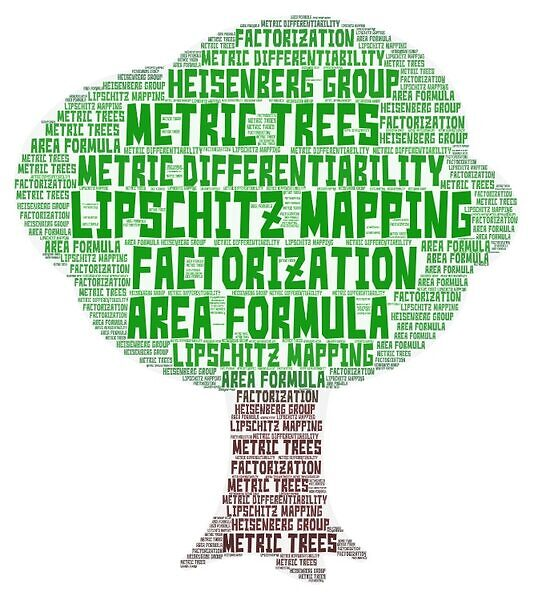# 2021 Summer Analysis on Metric Spaces Seminar### Date

Wednesday, August 11, 2021 - 09:00 to 10:00

Zoom

### Speaker: Piotr Hajlasz, University of Pittsburgh

Abstract: Given a Lipschitz map $f$ from a cube into a metric space, we find several equivalent conditions for $f$ to have a Lipschitz factorization through a metric tree. As an application we prove a recent conjecture of David and Schul. The techniques developed for the proof of the factorization result yield several other new and seemingly unrelated results. We prove that if $f$ is a Lipschitz mapping from an open set in $\mathbb{R}^n$ onto a metric space $X$, then the topological dimension of $X$ equals $n$ if and only if $X$ has positive $n$-dimensional Hausdorff measure. We also prove an area formula for length-preserving maps between metric spaces, which gives, in particular, a new formula for integration on countably rectifiable sets in the Heisenberg group. The talk is based on my recent joint paper with my graduate student Behnam Esmayli.